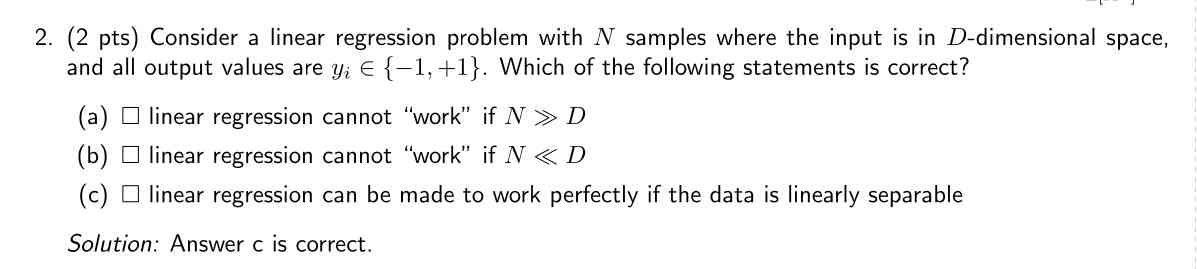### Linear separability for linear regression.

Dear TAs,

I am struggling to understand why linear separability matters for linear regression. If the data is linearly separable I understand how classification can benefit from it, however, I don't see how that can make regression "work" perfectly.

For example:
What if we have two variables (y,x) with a correlation of 0 that are perfectly linearly separable. How could this help to solve y = b0 + b1*x?I am not sure whether it is the case or not, but (a) and (b) appear wrong to me, because you can "compute" a regression in both cases, although it might not work very well depending on the setup.

I would say (c) is correct if we consider logistic regression, but indeed for linear regression, it is strange, because we want to minimize the MSE or MAE to a hyperplane...

I believe the use of the word "work" is a bad idea here, because it is not clear how to interpret it. Does it mean that we can find a plane that splits data perfectly ? Or does it mean we can find a hyperplane going through all the points, hence yielding a zero loss ? In the second case, we would need some data transformation, otherwise, since the labels are categorical, I believe it is not possible "as-is"

yes I am also confused, I thought linear regression couldn't work for N<<D because the matrix needed to find the w's wasn't invertible no?

That's a fair point, but a matrix not being invertible does not mean that you don't have a solution, you can have no or many, depending on the problem. For example, imagine having N = 1 and D = 3, it is easy to come up with a reasonable equation with a lot of solutions

I don't know if you find your answer to this finally, but this is finally just a classification problem as soon y takes only the values {-1,1}

yes but the MCQ doesn't say it is a classification problem it in fact specifically calls it 'linear regression problem' :/

Page 1 of 1